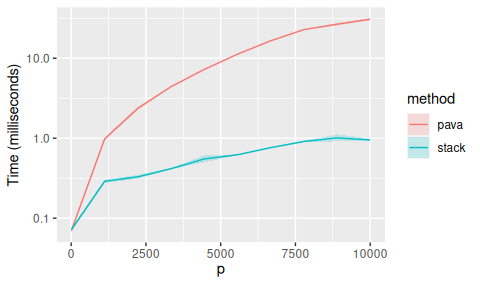# The Proximal Operator in SLOPE

The proximal operator for the sorted L1 norm, the penalty used in SLOPE, is defined as $\operatorname{prox}_J (v) = \operatorname*{arg\,min}_x\left( J(x; \lambda) + \frac 1 2 \lVert x - v \rVert_2^2 \right)$ where $$J(x; \lambda) = \sum_{j=1}^p \lambda_j |\beta_{(j)}|$$ is the sorted L1 norm, for which $|\beta_{(1)}| \geq |\beta_{(2)} \geq \cdots \geq |\beta_{(p)}.$

## Algorithms

There are several methods for solving this proximal operator and here we provide some benchmarks of these methods. Note that these results are almost entirely of academic nature and are added here only to serve as reference for others who are interested in working with SLOPE and particularly those who might be interested in improving the performance of these algorithms.

First, we load the packages we need,.

library(SLOPE)
library(tidyr)
library(dplyr)
library(bench)

Then we setup and run our benchmarks, letting p be the length of the vector used in the operator.

res <- expand_grid(
p = seq(10, 10000, length.out = 10),
i = 1:20,
method = c("stack", "pava"),
time = NA
)

set.seed(2254)

for (i in seq_len(nrow(res))) {
p <- res$p[i] x <- rnorm(p) lambda <- sort(runif(p), decreasing = TRUE) time <- bench_time(sortedL1Prox(x, lambda, res$method[i]))

res\$time[i] <- time[["real"]] * 1e3 # milliseconds
}

Finally, we summarize the results in the figure below.

library(ggplot2)
library(scales)

ggplot(res, aes(p, time, fill = method, col = method)) +
stat_summary(
geom = "ribbon",
fun.data = mean_se,
alpha = 0.2,
col = "transparent"
) +
stat_summary(geom = "line", fun = mean) +
scale_y_log10() +
labs(y = "Time (milliseconds)", x = expression(p))Comparison in execution times between the PAVA algorithm and the stack-based algorithm for solving the SLOPE prox.

As we can see, the stack-based algorithm appears to perform much better than the PAVA one does.